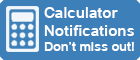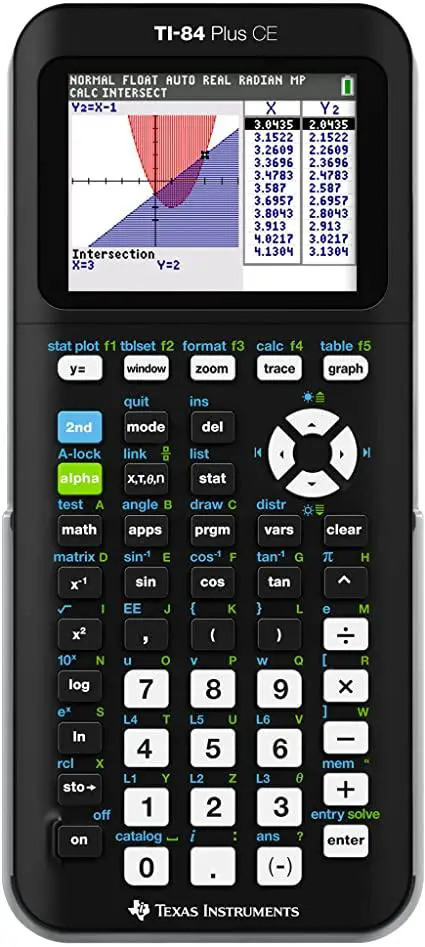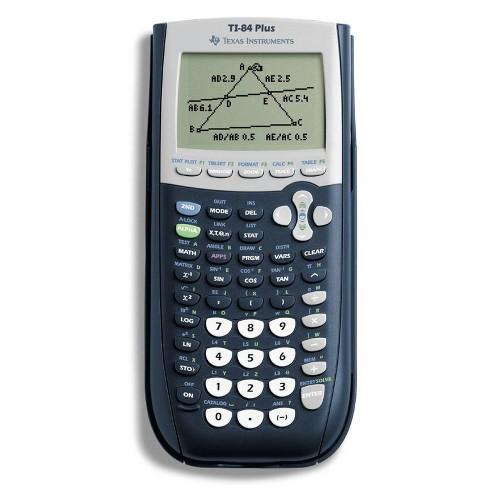Calculator Library
Free Online CalculatorsHome » 87 Calculators » Blog » Maximize Math Success With A TI-84 Calculator

Huge Domain Name Sale | Free Domains
Domain names on sale at crazy prices. Register a new domain for FREE when you buy another service.

## MAXIMIZE MATH SUCCESS WITH A TI-84 CALCULATORCalculators have been an essential tool for students for decades. They allow for quick and accurate computations, saving time and minimizing errors. With the ever-evolving technology, calculators have become even more advanced, offering various features and capabilities to enhance learning and problem-solving skills. One such calculator is the TI-84, which is widely used among students of multiple levels and subjects. In this article, we'll explore why every student should own a TI-84 calculator and the benefits it offers.

A TI-84 calculator is a graphing calculator produced by Texas Instruments. It's widely used in high school and college-level mathematics and science courses, including Algebra, Geometry, Trigonometry, Physics, Chemistry and Calculus. The TI-84 offers a simple interface with a clear, large display, making it easy to use.

The importance of calculators in students' education cannot be overstated. They provide a reliable tool for performing complex computations, making it easier for students to focus on understanding mathematical concepts rather than getting bogged down with arithmetic. Moreover, calculators can help students develop better problem-solving skills and improve test performance.

This article will discuss the advantages of the TI-84 calculator, how it enhances learning and its recommended uses. By the end of this article, we hope to provide a comprehensive overview of why every student should own a TI-84 calculator.

## Advantages Of The TI-84 Calculator

The TI-84 calculator offers numerous benefits to students, making it a valuable tool in their educational journey. Here are a few key advantages of the TI-84 calculator.

### User-friendly Interface

The TI-84 calculator features a simple and intuitive interface, making it easy for students to navigate and use. The large display is clear and easy to read, ensuring that students can accurately see the results of their computations. Additionally, the calculator's keypad is well-organized and labeled, minimizing the chances of mistakes and errors.

### Versatile Functionality

The TI-84 calculator is a versatile tool that can be used in various subjects, including Algebra, Geometry, Trigonometry, Physics, Chemistry and Calculus. Its graphing capabilities make it an excellent tool for visualizing mathematical concepts, helping students to understand and retain the information better.

### Graphing Capabilities

One of the most significant advantages of the TI-84 calculator is its graphing capabilities. The calculator can graph functions, perform statistical analysis and visualize geometric shapes, making it an essential tool for students in mathematics and science courses. This feature allows students to see patterns and relationships in data, making it easier to understand and analyze complex mathematical concepts.

### Storage and Memory

The TI-84 calculator has ample storage and memory, allowing students to store equations, formulas and other important information. This feature is especially beneficial for students who need to use the same information repeatedly or have a large amount of data to keep track of.

### Improved Test Performance

A calculator like the TI-84 can help students perform better on tests and exams. By allowing students to focus on understanding mathematical concepts rather than getting bogged down with arithmetic, the calculator helps them to function more effectively during assessments.

## How The TI-84 Calculator Enhances LearningThe TI-84 calculator isn't just a convenient tool for students; it's also a powerful tool that can enhance their learning and understanding of mathematical concepts. Here are a few ways the TI-84 calculator can help students in their studies.

### Visualization of Mathematical Concepts

One of the most significant advantages of the TI-84 calculator is its graphing capabilities. By allowing students to visualize mathematical concepts, the calculator helps them to understand and retain the information better. The calculator can graph functions, perform statistical analysis and visualize geometric shapes, making it an excellent tool for visualizing complex mathematical concepts.

### Improved Problem-Solving Skills

Using the TI-84 calculator can help students develop their problem-solving skills by allowing them to focus on the logic and reasoning behind mathematical computations. The calculator can perform complex calculations quickly and accurately, freeing up students' mental resources to concentrate on the thought process behind the calculations.

### Improved Test Performance

The TI-84 calculator can help students perform better on tests and exams by allowing them to focus on understanding mathematical concepts rather than getting bogged down with arithmetic. The calculator can perform complex computations quickly and accurately, allowing students to focus on the logic and reasoning behind the calculations and giving them more time to think critically about the material.

### Encourages Independent Learning

The TI-84 calculator is a self-sufficient tool that students can use to explore mathematical concepts independently. By allowing students to work through problems and concepts independently, the calculator encourages them to take an active role in their learning and helps build their confidence in their mathematical abilities.

## Recommended Uses Of The TI-84 CalculatorThe TI-84 calculator is a versatile tool that can be used in various ways to enhance students' learning and understanding of mathematical concepts. Here are a few recommended uses of the TI-84 calculator.

### Graphing Functions

One of the most powerful features of the TI-84 calculator is its graphing capabilities. The calculator can graph a range of functions, including linear, polynomial, exponential and logarithmic functions, making it an excellent tool for visualizing mathematical concepts. This feature is handy for students studying algebra, trigonometry and calculus.

### Statistical Analysis

The TI-84 calculator can also be used for statistical analysis, making it an excellent tool for students studying statistics. The calculator can perform a wide range of statistical computations, including mean, median, mode, standard deviation and correlation coefficients, allowing students to easily analyze and interpret data.

### Geometry

The TI-84 calculator is also an excellent tool for students studying geometry. The calculator can graph and manipulate geometric shapes, allowing students to visualize and understand geometric concepts. Additionally, the calculator can perform many geometric computations, including finding the area and perimeter of figures, solving triangles and calculating the volume of three-dimensional shapes.

### Calculus

The TI-84 calculator is an indispensable tool for students studying calculus. The calculator can perform various calculus computations, including finding derivatives, solving optimization problems and approximating definite integrals. With its powerful features, the TI-84 calculator is an excellent tool for helping students understand and retain calculus concepts.

## Conclusion

In conclusion, the TI-84 calculator is a must-have tool for every student. Its powerful features and versatility make it an excellent tool for enhancing students' learning and understanding of mathematical concepts. Whether used for graphing functions, statistical analysis, geometry, or calculus, the TI-84 calculator provides students with the tools they need to succeed.

In today's rapidly changing world, technology plays an increasingly important role in education. The TI-84 calculator is a prime example of how technology can enhance learning and make mathematical concepts more accessible to students. With its user-friendly interface and wide range of features, the TI-84 calculator is an excellent tool for students of all skill levels and backgrounds.

In short, every student should own a TI-84 calculator. With its powerful features and versatility, the TI-84 calculator provides students with the tools they need to succeed in their studies and beyond. Whether you're a student, educator, or simply someone who loves mathematics, the TI-84 calculator is an excellent tool that's sure to enhance your learning experience.

These resources provide a comprehensive overview of the TI-84 calculator and its features, its benefits for students and its role in enhancing learning and understanding of mathematical concepts. Whether you're a student, educator, or simply someone who loves mathematics, these resources provide a valuable reference that will enhance your understanding of the TI-84 calculator and its many uses.

 Previous Post« Essential Guide to Online Calculators Next PostTop 10 Calculator Myths »